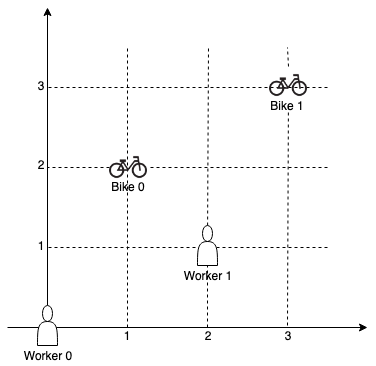# 1057. Campus Bikes

On a campus represented as a 2D grid, there are `N`workers and `M`bikes, with `N <= M`. Each worker and bike is a 2D coordinate on this grid.

Our goal is to assign a bike to each worker. Among the available bikes and workers, we choose the (worker, bike) pair with the shortest Manhattan distance between each other, and assign the bike to that worker. (If there are multiple (worker, bike) pairs with the same shortest Manhattan distance, we choose the pair with the smallest worker index; if there are multiple ways to do that, we choose the pair with the smallest bike index). We repeat this process until there are no available workers.

The Manhattan distance between two points `p1`and `p2`is `Manhattan(p1, p2) = |p1.x - p2.x| + |p1.y - p2.y|`. Return a vector `ans`of length `N`, where `ans[i]`is the index (0-indexed) of the bike that the `i`-th worker is assigned to.

Example 1:``````Input:
workers = [[0,0],[2,1]],
bikes = [[1,2],[3,3]]
Output:
[1,0]
Explanation:

Worker 1 grabs Bike 0 as they are closest (without ties), and Worker 0 is assigned Bike 1. So the output is [1, 0].
``````

Example 2:``````Input:
workers = [[0,0],[1,1],[2,0]],
bikes = [[1,0],[2,2],[2,1]]
Output:
[0,2,1]
Explanation:

Worker 0 grabs Bike 0 at first. Worker 1 and Worker 2 share the same distance to Bike 2, thus Worker 1 is assigned to Bike 2, and Worker 2 will take Bike 1. So the output is [0,2,1].
``````

Note:

1. `0 <= workers[i][j], bikes[i][j] < 1000`
2. All worker and bike locations are distinct.
3. `1 <= workers.length <= bikes.length <= 1000`

Thoughts:

1. number of workers and bikes is bounded -> Bucket sort Pigeonhole sort: buckets[i]: a list with <worker id, bike id> pair with distance i (Original post, Java post)
2. Priority queue when number of workers and bikes is not bounded (Original post, Python post)

Code: Bucket Sort T: O(M *N), S: O(M * N)

``````class Solution {
public:
vector<int> assignBikes(vector<vector<int>>& workers, vector<vector<int>>& bikes) {
vector<vector<pair<int, int >>> bucket(2001);

// T: O(M * N); S: O(M * N)
// range of keys is known: [0, 2000] -> key -> (worker id, bike id)
int n = workers.size(), m = bikes.size();
for (int i = 0; i < n; i ++){
for (int j = 0; j < m; j ++){
int dis = abs(workers[i] - bikes[j]) + abs(workers[i] - bikes[j]);
bucket[dis].push_back({i,j});
}
}

vector<int> ans(n, -1);
vector<bool> bikeUsed (m, false);

for (int d = 0; d <= 2000; d ++){
for (int k = 0; k < bucket[d].size(); k++){
if (ans[bucket[d][k].first] == -1 && !bikeUsed[bucket[d][k].second]){
bikeUsed[bucket[d][k].second] = true;
ans[bucket[d][k].first] = bucket[d][k].second;
}
}
}

return ans;

}
};
``````

Code: ~ Java

``````public int[] assignBikes(int[][] workers, int[][] bikes) {
List<int[]>[] buckets = new List;
for (int i = 0; i < workers.length; i++) {
for (int j = 0; j < bikes.length; j++) {
int dis = Math.abs(bikes[j] - workers[i]) + Math.abs(bikes[j] - workers[i]);
if (buckets[dis] == null) {
buckets[dis] = new ArrayList<>();
}
buckets[dis].add(new int[] {i, j});
}
}
int[] res = new int[workers.length];
Arrays.fill(res, -1);
Set<Integer> assignedBike = new HashSet<>();
for (int i = 0; i < buckets.length && assignedBike.size() < workers.length; i++) {
if (buckets[i] != null) {
for (int[] pair : buckets[i]) {
if (res[pair] < 0 && !assignedBike.contains(pair)) {
res[pair] = pair;
}
}
}
}
return res;
}
``````

Code: PQ T: O(nlogn)

``````class Solution {
public int[] assignBikes(int[][] workers, int[][] bikes) {
int w = workers.length, b = bikes.length;

int [] wo = new int[w], bi = new int [b];
Arrays.fill(wo, -1);
Arrays.fill(bi, -1);
PriorityQueue<int []> pq = new PriorityQueue<>(new Comparator<int[]>(){
@Override
public int compare(int [] a, int [] b){
return a != b ? a - b
: (a != b ? a - b
: (a - b));
}
});

// push into pq
for (int i = 0; i < w; i ++){
for(int j = 0; j < b; j++){
int[] worker = workers[i], bike = bikes[j];
int dist = Math.abs(worker - bike) + Math.abs(worker - bike);
pq.offer(new int []{dist, i, j});
}
}

// retrieve the ans
int assigned = 0;
while(!pq.isEmpty() && assigned < w){
int [] entry = pq.poll();
if (wo[entry] == -1 && bi[entry] == -1){
wo[entry] = entry;
bi[entry] = entry;
assigned++;
}
}

return wo;
}
}
``````

Code: ~ Python

``````    def assignBikes(self, workers, bikes):
distances = []     # distances[worker] is tuple of (distance, worker, bike) for each bike
for i, (x, y) in enumerate(workers):
distances.append([])
for j, (x_b, y_b) in enumerate(bikes):
distance = abs(x - x_b) + abs(y - y_b)
distances[-1].append((distance, i, j))
distances[-1].sort(reverse = True)  # reverse so we can pop the smallest distance

result = [None] * len(workers)
used_bikes = set()
queue = [distances[i].pop() for i in range(len(workers))]   # smallest distance for each worker
heapq.heapify(queue)

while len(used_bikes) < len(workers):
_, worker, bike = heapq.heappop(queue)
if bike not in used_bikes:
result[worker] = bike
else:
heapq.heappush(queue, distances[worker].pop())  # bike used, add next closest bike

return result
``````

Fewer lines:

``````class Solution:
def assignBikes(self, W: List[List[int]], B: List[List[int]]) -> List[int]:
ans, used = [-1] * len(W), set()
for d, w, b in sorted([abs(W[i] - B[j]) + abs(W[i] - B[j]), i, j] for i in range(len(W)) for j in range(len(B))):
if ans[w] == -1 and b not in used:
ans[w] = b